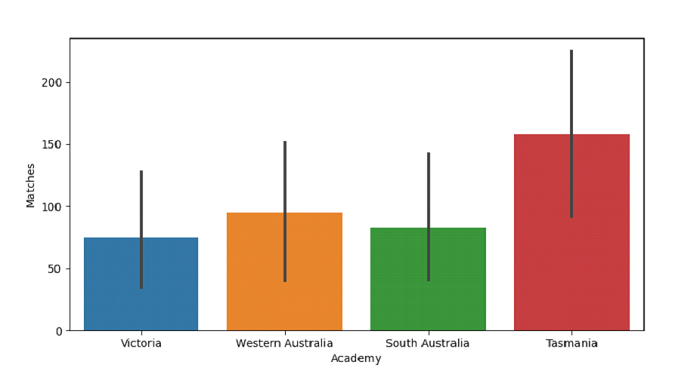# Python Pandas - Draw a Bar Plot and control swarm order by passing an explicit order with Seaborn

Bar Plot in Seaborn is used to show point estimates and confidence intervals as rectangular bars. The seaborn.barplot() is used. Control ordering by passing an explicit order i.e. ordering on the basis of a specific column using the order parameter.

Let’s say the following is our dataset in the form of a CSV file −Cricketers2.csv

At first, import the required libraries −

import seaborn as sb
import pandas as pd
import matplotlib.pyplot as plt

Load data from a CSV file into a Pandas DataFrame −

dataFrame = pd.read_csv("C:\Users\amit_\Desktop\Cricketers2.csv")


Plotting horizontal bar plots with Matches and Academy columns. Control order by passing an explicit order i.e. ordering on the basis of "Academy" using the order parameter −

sb.barplot(x = dataFrame["Academy"], y = dataFrame["Matches"],order = ["Victoria", "Western Australia", "South Australia", "Tasmania"])

## Example

Following is the code −

import seaborn as sb
import pandas as pd
import matplotlib.pyplot as plt

# Load data from a CSV file into a Pandas DataFrame

# plotting horizontal bar plots with Matches and Academy
# Control order by passing an explicit order i.e. ordering on the basis of "Academy" using order parameter
sb.barplot(x = dataFrame["Academy"], y = dataFrame["Matches"],order = ["Victoria", "Western Australia", "South Australia", "Tasmania"])

# display
plt.show()

## Output

This will produce the following output −Updated on: 01-Oct-2021

90 Views